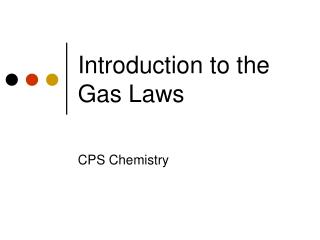DownloadDownload PresentationIntroduction to the Gas Laws

# Introduction to the Gas Laws

Télécharger la présentation## Introduction to the Gas Laws

- - - - - - - - - - - - - - - - - - - - - - - - - - - E N D - - - - - - - - - - - - - - - - - - - - - - - - - - -
##### Presentation Transcript

1. Introduction to the Gas Laws CPS Chemistry

2. What is a Gas? • A state of matter where there is indefinite volume (will fill its container) and indefinite density (can be compressed) • Gasses are fluids, because like liquids they have the ability to flow • Gasses are made up of randomly moving molecules, but are mostly empty space

3. Temperature • Temperature is the measure of how hot or cold an object is relative to something else • Temperature in a gas relates to the kinetic energy of the molecules, the hotter the temperature, the faster they move • Temperature in gas law problems is always measured Kelvin, convert all temps to K • Standard temperature is 273K

4. Pressure • Pressure is force/area, N/m2 or a Pascal • Pressure is directly proportional to applied force • Pressure is indirectly proportional to area • Pressure in gasses is the result of the number of collisions that the molecules make with the container, so the greater the temperature, the greater the pressure

5. Pressure cont. • Units of pressure are: torr mm of Hg (millimeters of mercury) atm (atmospheres) • Standard Pressure is: 760 torr 760 mm of Hg 1 atmosphere

6. Volume • Volume is the amount of space that gasses take up, gasses will fully fill any container that they are in • Two units of volume are Cubic centimeters: cc or cm3 Millileters: mL 1 cc = 1 cm3 = 1 mL

7. Boyles Law • Pressure and volume are inversely proportional, if you increase one, you decrease the other citation

8. The equation P1V1 = P2V2 This works when the temperature is kept constant. Before you start working with ANY gas law problem, make sure that you have all the same units!

9. Example 1 • You have 2.0L of hydrogen gas at 3.0 atmospheres, you increase the pressure to 12.0 atm, what is your new volume?

10. Example 2 • You have 30cm3 of chlorine gas at 1000 torr you change the volume to 90cm3 what is your new pressure? (solve for torr, mm of Hg and atm)

11. Charles Law • When pressure is kept constant the relationship between Temperature and volume is citation

12. V1 V2 = T1 T2 The Formula • The formula is

13. Example 1 • If pressure is held constant, and a 2.0L of Neon gas is heated from 0°C to 100°C, what is the new volume?

14. Example 2 • If pressure is held constant, and a 850cc of Argon gas is cooled from 50°C to 10°C, what is the new volume?

15. Gay-Lussac’s Law • “The pressure of a fixed mass of gas at a constant volume varies directly with the temperature (K).” • If you have a certain amount of gas in a fixed sized container, the pressure will change if the temperature changes.

16. The formula P1= P2 T1 T2

17. Example 1 A cylinder of compressed gas has a pressure of 4.882 atm on one day. The next day the same cylinder of gas has a pressure of 4.690 atm and its temperature is 8°C. What was the temperature on the previous day in C?

18. Example 2 The safety limit for a certain can of aerosol stay is 95°C. If the pressure of the gas in the can is 2.96 atm when it is 17°C, what will the pressure be at the safety limit?

19. Combined Gas Law • When you have all three variables involved (Pressure, Temperature, and Volume) you use the combined gas law P1V1 P2V2 = T1 T2

20. Example 1 • A 50 mL sample of fluorine gas at 15°C that rises in temperature to 80°C, if the original pressure is 2 atm and increases to 8 atm, what is the new volume?

21. Example 2 • A 500 cc sample of boron gas at 85°C that cools to 0°C, if the original pressure is 768 torr and increases to 850 torr what is the new volume?

22. Partial Pressures • The total pressure in a combination of gasses is the sum of all the pressures of each individual gas. Ptot=P1+P2+P3… *all gas pressures must be in the same unit

23. Example 1 • The total pressure for air in a scuba tank is 1200 torr, if the oxygen has a pressure of 500 torr, the Nitrogen is 350 torr, what is the pressure of the argon gas component?

24. Ideal Gas Law • All the prior gas laws involve a combination of pressure, temperature, or volume. The ideal gas law also takes into account the number of molecules of gas involved. PV=nRT

25. Ideal gas law cont. P= pressure = atmospheres V = volume = Liters T = temperature = Kelvin n = number of moles = mol R = gas constant = .0821 L * atm mol * K

26. Example 1 • A rigid cylinder with a volume of 10.0 liters is filled with neon gas at a pressure of 250. atm at 30°C, how many moles are in the gas?

27. Example 2 • A 12.0 liter flask at 45°C has 5.00 moles of helium gas, what is the pressure?